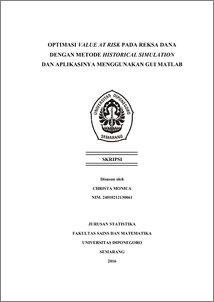# OPTIMASI VALUE AT RISK PADA REKSA DANA DENGAN METODE HISTORICAL SIMULATION DAN APLIKASINYA MENGGUNAKAN GUI MATLAB

MONICA, CHRISTA (2016) OPTIMASI VALUE AT RISK PADA REKSA DANA DENGAN METODE HISTORICAL SIMULATION DAN APLIKASINYA MENGGUNAKAN GUI MATLAB. PhD thesis, FSM Universitas Diponegoro.Preview
PDF
4Mb

## Abstract

Value at Risk (VaR) is a method used to measure financial risk within a firm or investment portfolio over a specific time period at certain confidence interval level. Historical Simulation is used in this research to compute VaR of stock mutual fund at 5% confidence interval level, with one day time period and Rp 100.000.000,00 startup investment fund. Historical Simulation ia a non parametric method where the formula doesn’t require any asumption. Portfolio optimization is done by calculating the weight of allocation fund for each asset in the portfolio using Mean Variance Efficient Portfolio (MVEP) method. The data in this research are divided into four mutual fund asset. To make VaR become easier for people to understand, an application is made using GUI in Matlab. The smallest risk value for single investment asset is obtained by Valbury Equity I stock mutual fund and the smallest risk value for two-asset portfolio is obtained by the combination assets of Pacific Equity Fund and Valbury Equity I. Meanwhile for three-asset portfolio, the combination assets of Pacific Equity Fund, Valbury Equity I, and Millenium Equity Prima Plus have the smallest risk value. The test result of VaR with Basel Rules shows that the usage of VaR is legitimate to measure loses potency in mutual fund investment. Keywords: Value at Risk (VaR), Historical Simulation, Mutual Fund, Risk.

Item Type: Thesis (PhD) H Social Sciences > HA Statistics Faculty of Science and Mathematics > Department of Statistics 49859 Mr Hasbi Yasin 24 Aug 2016 15:33 24 Aug 2016 15:33

Repository Staff Only: item control page